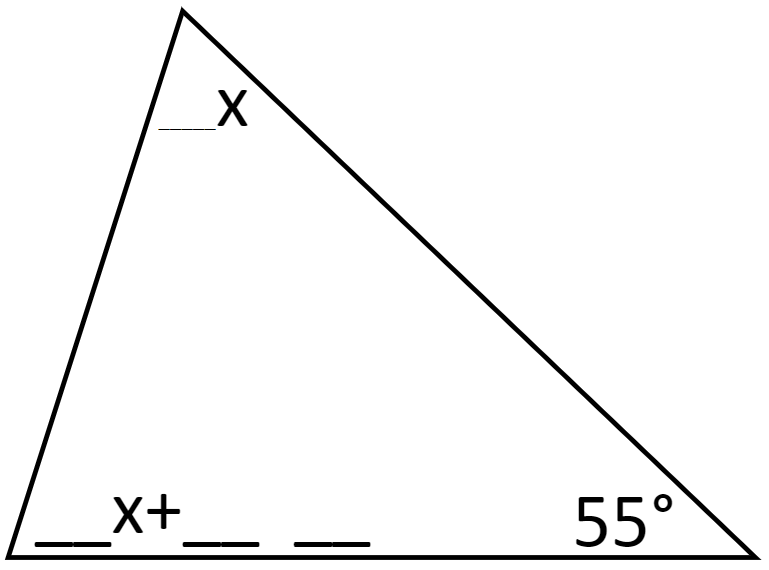Home > Grade 8 > Geometry > Triangle Sum Theorem

# Triangle Sum Theorem

Directions: Using the digits 1-9, at most one time each, to fill in the blanks. Then solve the problem for x. Choose numbers such that the solution for x is a whole number.### Hint

How many degrees must the two unknown angles sum to?
Can you write an equation to represent the sum of the two unknown angles?

There are many answers. One is:
Top angle is 4x and bottom angle is 6x + 25.
x = 10 solves the problem.

Extension/extra challenge 1: set a value for x and find all the ways to make the problem have that solution.
Extension/extra challenge 2: change the value of the bottom right angle.

Source: Franco D. Adkins

## Square Root Expression

Directions: Use the digits 1 to 9, at most one time each, to fill in …